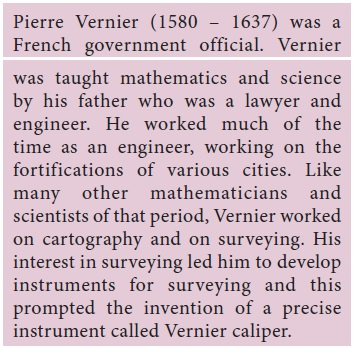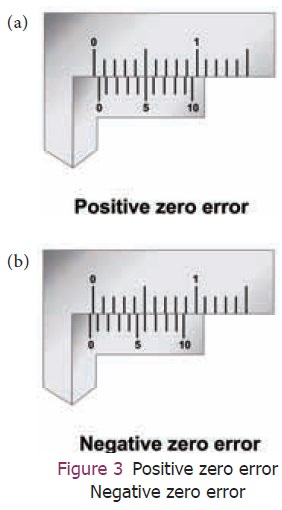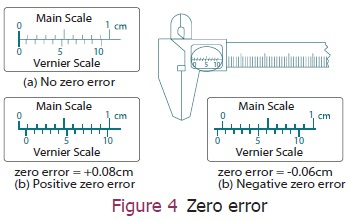Home | | Science 9th std | Vernier caliper and Screw Gauge

# Vernier caliper and Screw Gauge

In our daily life, we use metre scale for measuring lengths. They are calibrated in cm and mm scales. The smallest length which can be measured by metre scale is called least count.

Vernier caliper and Screw Gauge

In our daily life, we use metre scale for measuring lengths. They are calibrated in cm and mm scales. The smallest length which can be measured by metre scale is called least count. Usually the least count of a scale is 1 We can measure the length of objects up to mm accuracy using this scale. But this scale is not sufficient for measuring the size of small spherical objects. So, Vernier caliper and screw gauge are used.## 1. Vernier scale

The diameters of spherical objects such as cricket ball and hollow objects such as a pen cap cannot be measured with a meter scale. For that we use an instrument named Vernier caliper which can measure the inner and outer diameters of objects.## 2. Description of Vernier caliper

The Vernier caliper consists of a thin long steel bar graduated in cm and mm. This is the main scale. To the left end of the steel bar an upper and a lower jaw are fixed perpendicular to the bar.These are named as fixed jaws. To the right of the fixed jaws, a slider with an upper and a lower moveable jaw is fixed. The slider can be moved or fixed to any position using a screw. The Vernier scale is marked on the slider and moves along with the movable jaws and the slider. The lower jaws are used to measure the external dimensions and the upper jaws are used to measure the internal dimensions of objects. The thin bar attached to the right side of the Vernier scale is used to measure the depth of hollow objects.

## 3. Usage of Vernier caliper

The first step in using the Vernier caliper is to find out its least count, range and zero error.

### Least countThe main scale division can easily be obtained by inspecting the main scale. It will be in centimeter, further divided into millimetre. The value of the smallest main scale division is 1 mm. The Vernier scale division is obtained by counting number of division in it. In the Vernier scale there will be 10 divisions.### Zero error

Unscrew the slider and move it to the left, such that both the jaws touch each other. Check whether the zero marking of the main scale coincides with that of the Vernier scale. If they are not coinciding with each other, the instrument is said to posses zero error. Zero error may be positive or negative. If the zero mark of the Vernier is shifted to the right, it is called positive error. On the other hand, if the Vernier zero is shifted to the left of the main scale zero marking, then the error is negative.

### Positive zero error

Figure 3(a) shows the positive zero error. From the figure you can see that zero of the vernier scale is shifted to the right of zero of the main scale. In this case the reading will be more than the actual reading. Hence, this error should be corrected. In order to correct this error, find out which vernier division is coinciding with any of the main scale divisions. Here, fifth vernier division is coinciding with a main scale division. So, positive zero error = +5 x LC = +5 x 0.01 = 0.05 cm.### Negative zero error

Now look at the Figure 3(b). You can see that zero of the vernier scale is shifted to the left of the zero of the main scale. So, the obtained reading will be less than the actual reading. To correct this error we should first find which vernier division is coinciding with any of the main scale divisions, as we found in the previous case. In this case, you can see that sixth line is coinciding. But, to find the negative error, we can count backward (from 10). So, the fourth line is coinciding. Therefore, negative zero error = ‒4×LC = ‒4×0.01 = ‒0.04 cm.

#### Example:

Calculate the positive and negative error from the given Figure 4.Solution:

Case (a): Zero of the vernier scale and zero of the main scale are coinciding with each other. So there is no zero error.

Case (b): e zero of veriner scale is shi ed to the right from the zero of the main scale. It is positive error. The 8th division of vernier scale coincides with one of the main scale divisions. So the positive error = (8×0.01cm) = 0.08 cm.

Case (c): e zero of veriner scale is shi ed to the le from the zero of main scale. It is negative error. The 4th division of vernier scale (6th from backward) coincides with one of the main scale divisions. So the negative zero error = ‒(6×0.01cm)= ‒0.06 cm.

Once you are able to calculate the zero error, you can get the correct reading using the formula:

The correct reading = Main scale reading + (VC × LC) ± (Zero correction)

### Zero Correction:

If error is positive the we should subtract that error value. If error is negative, we should add that error value.

For example, let us calculate the correct reading, if the main scale reading is 8 cm, vernier coincidence is 4 and positive zero error is 0.05 cm,

The correct reading = 8 cm + (4 × 0.01cm) – 0.05 cm = 8 + 0.04 – 0.05 = 8 – 0.01 = 7.99 cm

Let us try another one. The main scale reading is 8 cm and vernier coincidence is 4 and negative zero error is 0.02 cm, then the correct reading:

=8 cm+(4×0.01 cm)+(0.02 cm)

=8+0.04+0.02 = 8.06 cm.

We can use Vernier caliper to nd different dimensions of any familiar object. If the length, width and height of the object can be measured, volume can be calculated. For example, if we could measure the inner diameter of a beaker (using appropriate jaws) as well as its depth (using the depth probe) we can calculate its inner volume.

#### Example:

Calculate the diameter of the sphere which is shown in the Figure 5. Assume the scale has no zero error.The diameter (D) of the sphere = Main scale reading (MSR) + (Vernier scale coincidence (VC) × least count (LC))± ZE. In this case the zero of the vernier scale is right after the main scale reading 1.1. So the main scale reading is 1.1 cm. The vernier scale coincidence is 7. The least count is 0.01 cm. The diameter of the sphere = 1.1 cm + (7 × 0.01cm)‒0 = 1.1 + 0.07 = 1.17 cm

## 4. Digital Vernier caliper

Today, we are living in a digital world and the digital version of the vernier callipers are available now. Digital Vernier caliper (Figure 6) has a digital display on the slider, which calculates and displays the measured value. The user need not manually calculate the least count, zero error etc.Tags : Measuring Instruments , 9th Science : Measurement and Measuring Instruments
Study Material, Lecturing Notes, Assignment, Reference, Wiki description explanation, brief detail
9th Science : Measurement and Measuring Instruments : Vernier caliper and Screw Gauge | Measuring Instruments